• 编译原理语义分析和中间代码生成实验报告，基于VS2010开发的纯C#的程序，附程序执行截图
• 重点：语义分析的两个作用 <3> 语义分析的方法 2. 中间代码 重点：要求中间代码具有如下特性，以便于编译器的开发移植和代码的优化： 3.后缀式 定义 算法实现 4.后缀式的计算 5.三地址码 6.四元式主要由四部分组成：...
文章目录1. 语义与语法的区别重点：语义分析的两个作用<3> 语义分析的方法2. 中间代码重点：要求中间代码具有如下特性，以便于编译器的开发移植和代码的优化：3.后缀式定义算法实现4.后缀式的计算5.三地址码6.四元式主要由四部分组成：三地址代码例题有文法 G 和 G 的语法制导翻译如下：7.符号表8. 数组元素的引用9. 布尔表达式10. 控制语句11.过程的定义与声明左值和右值拉链回填
1. 语义与语法的区别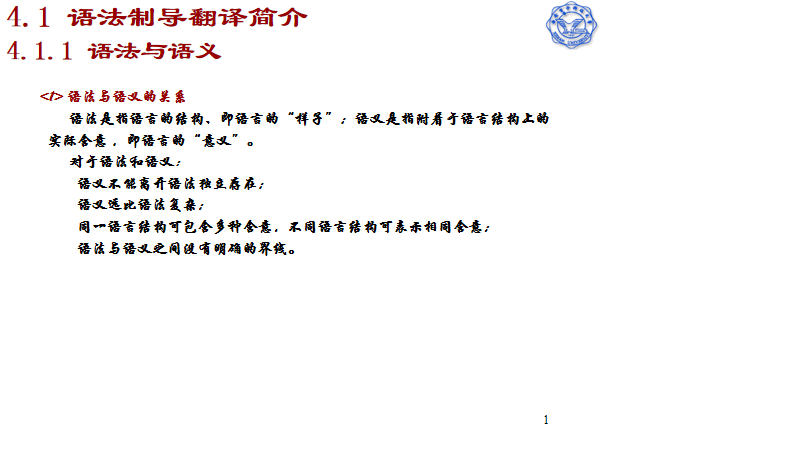<1> 语法与语义的关系
语法是指语言的结构、即语言的“样子”；语义是指附着于语言结构上的实际含意 ，即语言的“意义”。
对于语法和语义：
语义不能离开语法独立存在；
语义远比语法复杂；
同一语言结构可包含多种含意，不同语言结构可表示相同含意；
语法与语义之间没有明确的界线。
重点：语义分析的两个作用
1.检查是否结构正确的句子所表示的意思也合法；
2.执行规定的语义动作，如：
表达式求值
符号表填写
中间代码生成等
<3> 语义分析的方法
语法制导翻译
2. 中间代码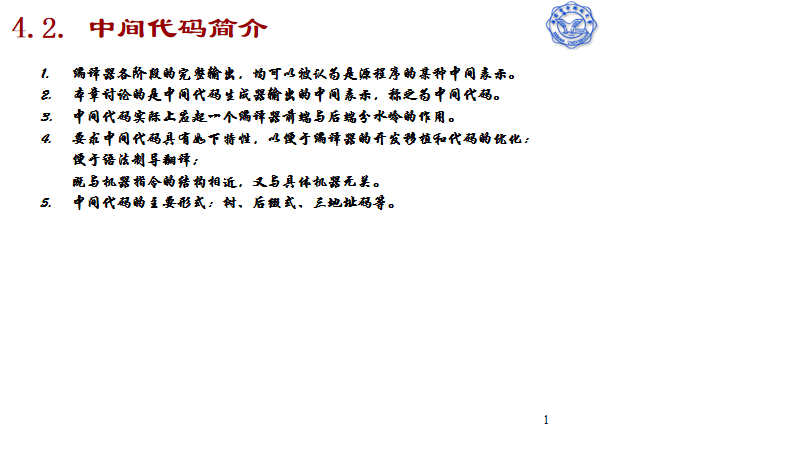重点：要求中间代码具有如下特性，以便于编译器的开发移植和代码的优化：
便于语法制导翻译；
既与机器指令的结构相近，又与具体机器无关。
3.后缀式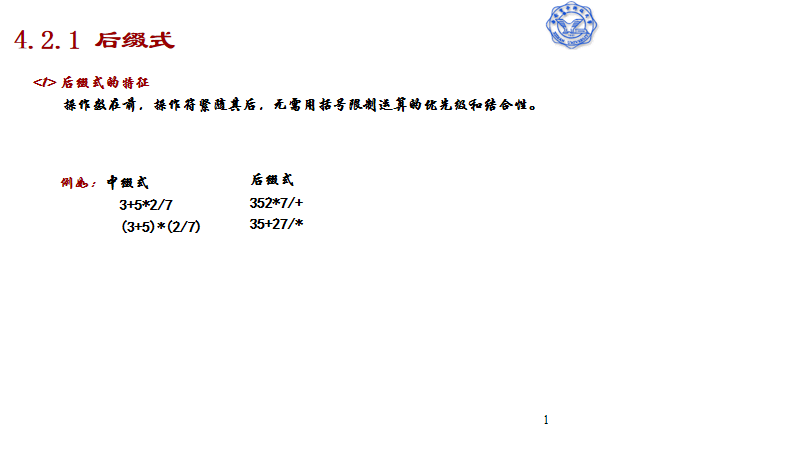定义
一个表达式E的后缀形式可以如下定义：
（1）如果E是一个变量或常量，则E的后缀式是E本身。
（2）如果E是E1 op E2形式的表达式，这里op是任何二元操作符，则E的后缀式为E1’E2’ op，这里E1’和E2’分别为E1和E2的后缀式。
（3)如果E是（E1）形式的表达式，则E1的后缀式就是E的后缀式。
如：我们平时写a+b，这是中缀表达式，写成后缀表达式就是：ab+
(a+b)c-(a+b)/e的后缀表达式为：
(a+b)c-(a+b)/e
→((a+b)c)((a+b)/e)-
→((a+b)c)((a+b)e/)-
→(ab+c)(ab+e/)-
→ab+cab+e/-
算法实现
将一个普通的中序表达式转换为逆波兰表达式的一般算法是：
首先需要分配2个栈，一个作为临时存储运算符的栈S1（含一个结束符号），一个作为输入逆波兰式的栈S2（空栈），S1栈可先放入优先级最低的运算符#，注意，中缀式应以此最低优先级的运算符结束。可指定其他字符，不一定非#不可。从中缀式的左端开始取字符，逐序进行如下步骤：
（1）若取出的字符是操作数，则分析出完整的运算数，该操作数直接送入S2栈
（2）若取出的字符是运算符，则将该运算符与S1栈栈顶元素比较，如果该运算符优先级(不包括括号运算符)大于S1栈栈顶运算符优先级，则将该运算符进S1栈，否则，将S1栈的栈顶运算符弹出，送入S2栈中，直至S1栈栈顶运算符低于（不包括等于）该运算符优先级，最后将该运算符送入S1栈。
（3）若取出的字符是“（”，则直接送入S1栈顶。
（4）若取出的字符是“）”，则将距离S1栈栈顶最近的“（”之间的运算符，逐个出栈，依次送入S2栈，此时抛弃“（”。
（5）重复上面的1~4步，直至处理完所有的输入字符
（6）若取出的字符是“#”，则将S1栈内所有运算符（不包括“#”），逐个出栈，依次送入S2栈。
完成以上步骤，S2栈便为逆波兰式输出结果。不过S2应做一下逆序处理。便可以按照逆波兰式的计算方法计算了！
4.后缀式的计算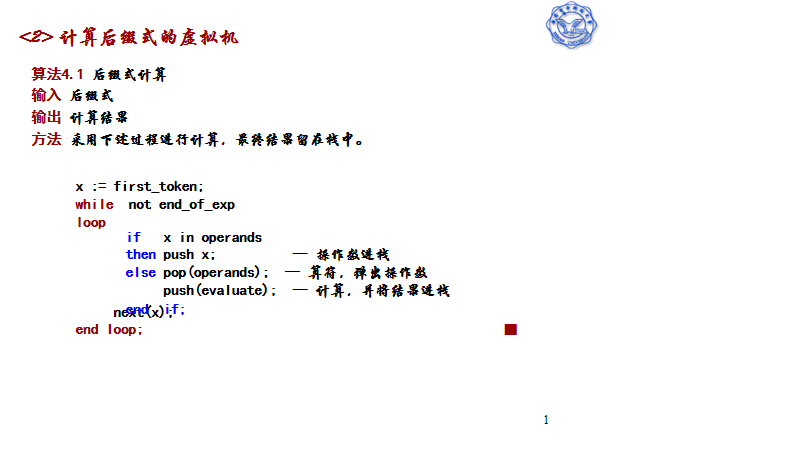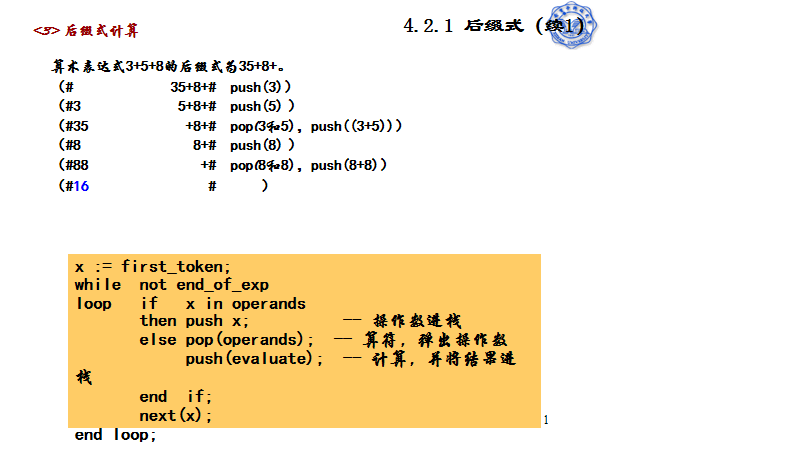5.三地址码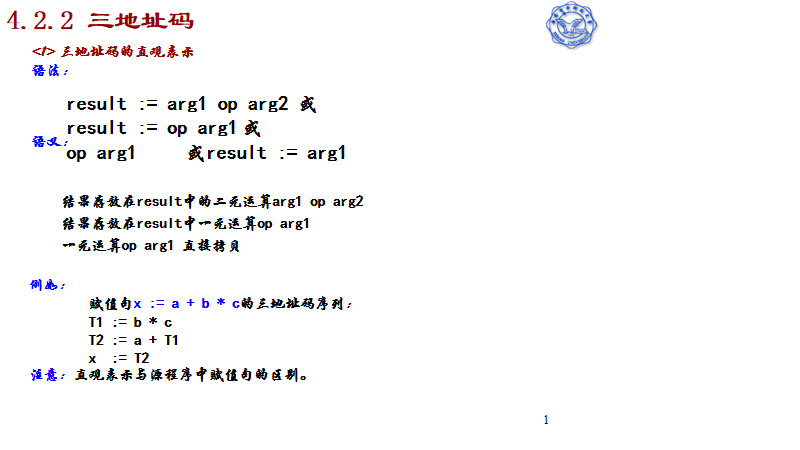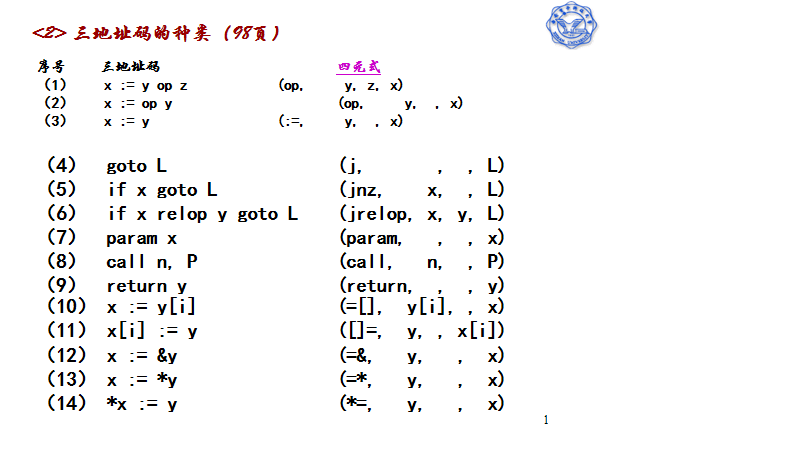6.四元式主要由四部分组成：
（OP，arg1，arg2，result）
其中，OP是运算符，argl，arg2分别是第一和第二个运算对象，result是编译程序为存放中间运算结果而引进的变量，常称为临时变量。当OP是一目运算时，常常将运算对象定义为arg1。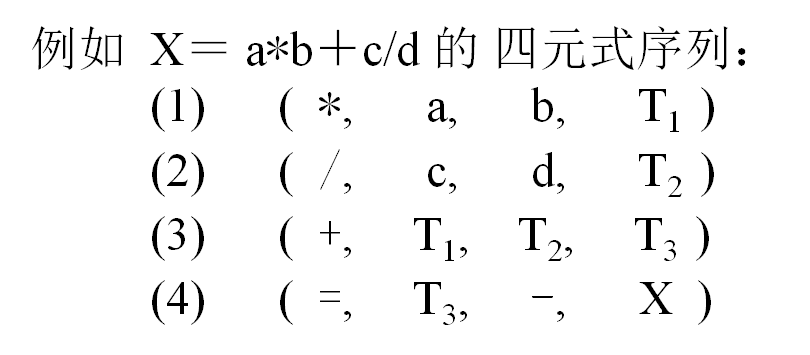四元式出现的顺序和语法成份的计值顺序相一致。
四元式之间的联系是通过临时变量实现的，这样易于调整和变动四元式。
便于优化处理。
三地址代码
三地址代码是四元式的另一种表示形式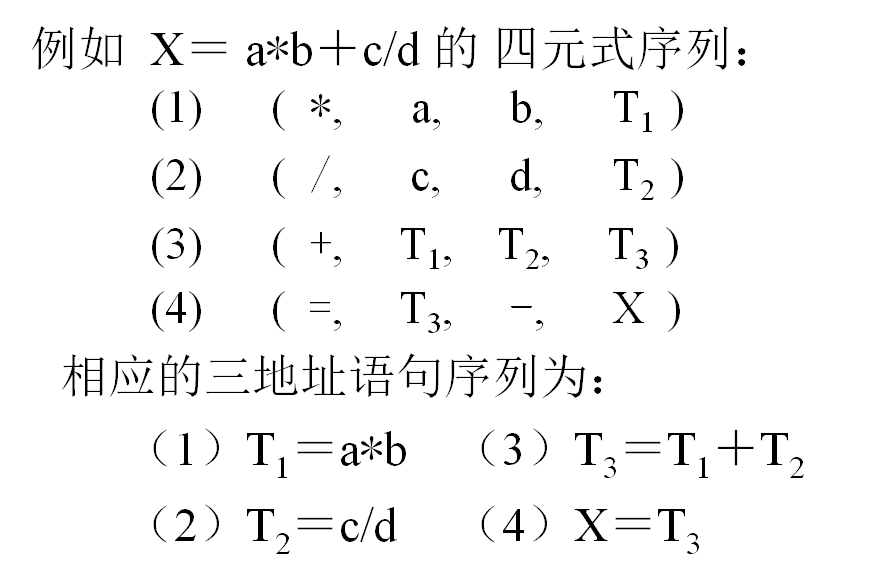例题有文法 G 和 G 的语法制导翻译如下：
E → E1*T { E.place=newtemp;
emit(*,E1.place,T.place,E.place; }
| T   { E.place=T.place; }
T → T1+F { T.place=newtemp;
emit(+,T1.place,F.place,T.place; }
| F   { T.place=F.place; }
F → (E)    { F.place=E.place; }
| id   { F.place=id.name; }
(a) 求句型 (T+F)*id 的短语、直接短语以及句柄；
(b) 根据语法制导翻译写出句子 ab+cd 的中间代码；
© （若 a=3 ， b=5 ， c=7 ， d=8 ，请给出中间代码计算结果；
(d) 将文法 G 简化为： E → E*T|T ， T → T+F|F ， F → id 。给出它的识别活前缀的 DFA 。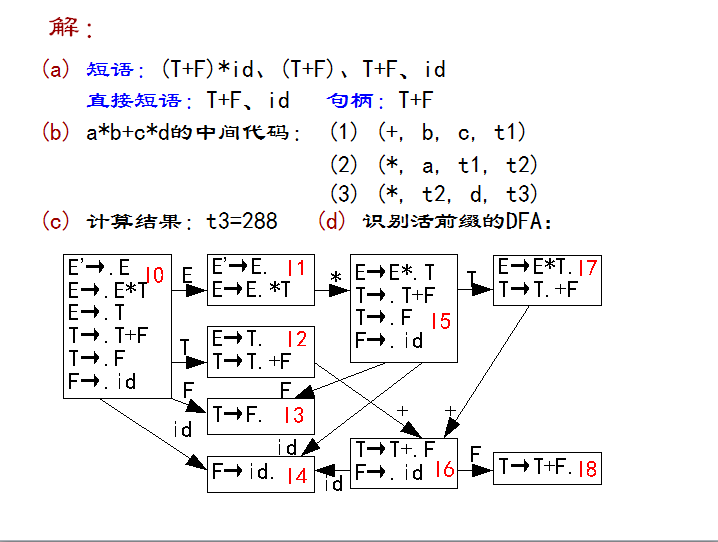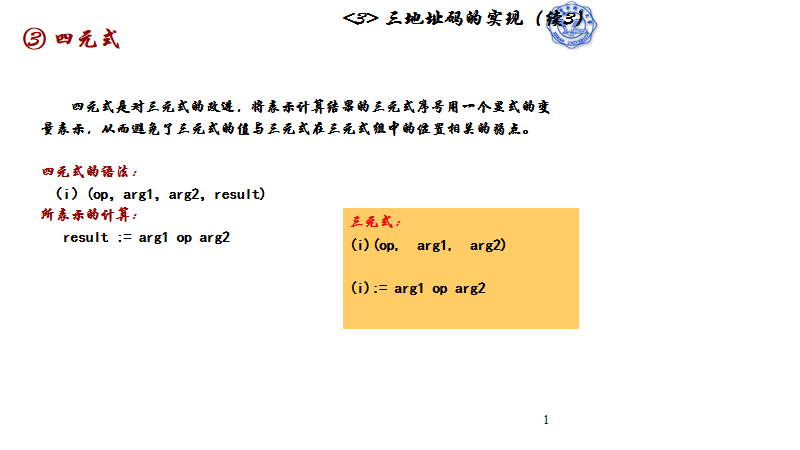7.符号表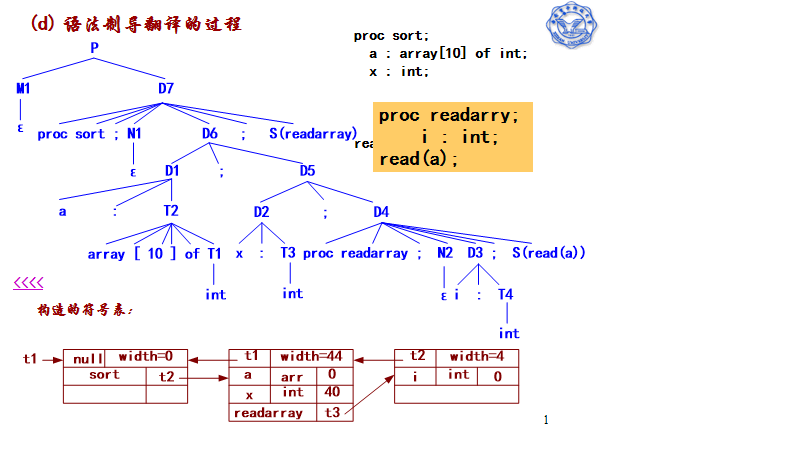8. 数组元素的引用
（1）数组元素的地址计算
注意是行主存储还是列主存储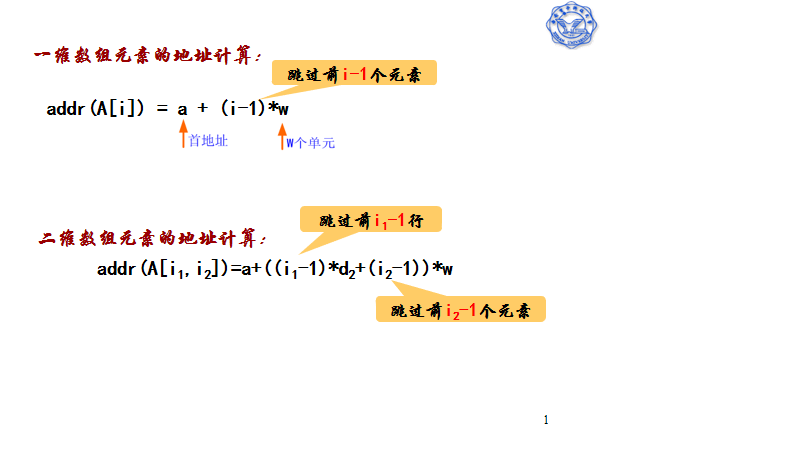（2）☆数组元素引用的语法制导翻译(考试热点之一)
9. 布尔表达式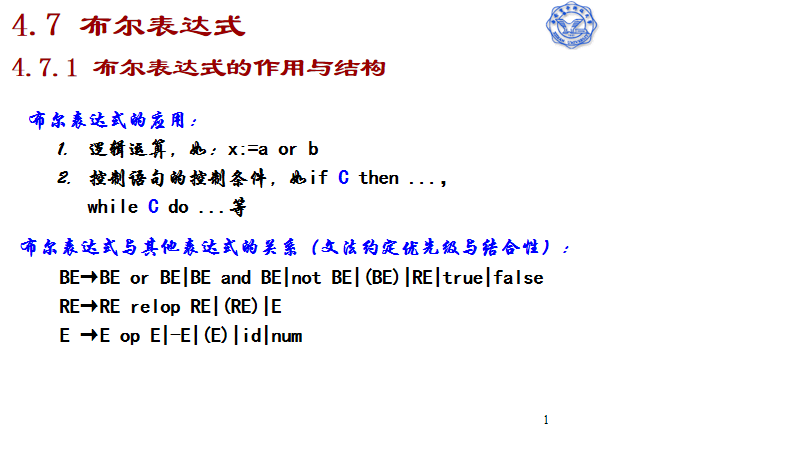布尔表达式的计算有两种方法：数值表示的直接计算和逻辑表示的短路计算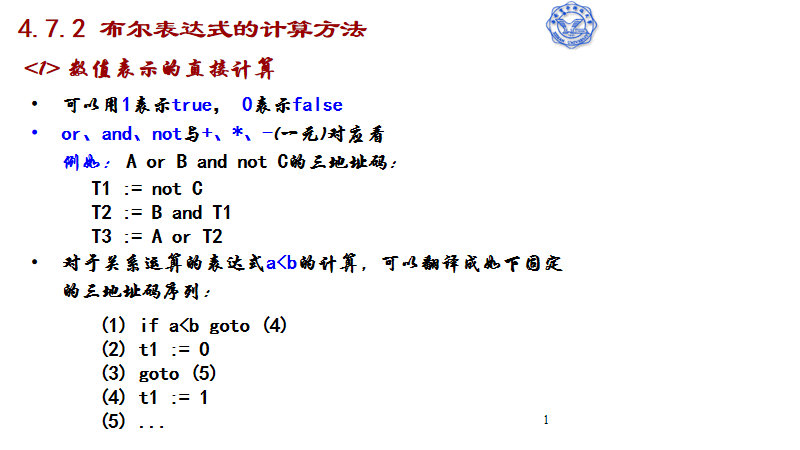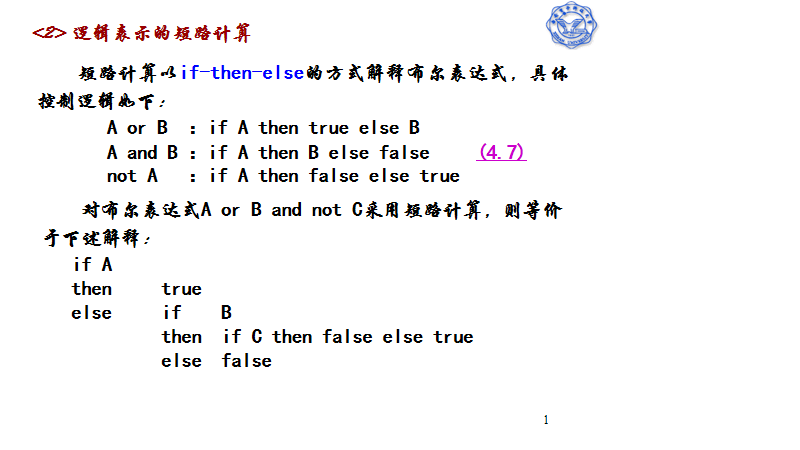☆ 布尔表达式短路计算的翻译：短路计算的控制流，真出口与假出口，真出口链与假出口链，拉链回填技术(P207 例4.41)（考试热点之一）
10. 控制语句
控制语句的分类：①无条件转移、②条件转移、③循环语句、④分支语句
无条件转移(goto)\条件转移(if、while)
条件转移的语法制导翻译：P213 例4.42
11.过程的定义与声明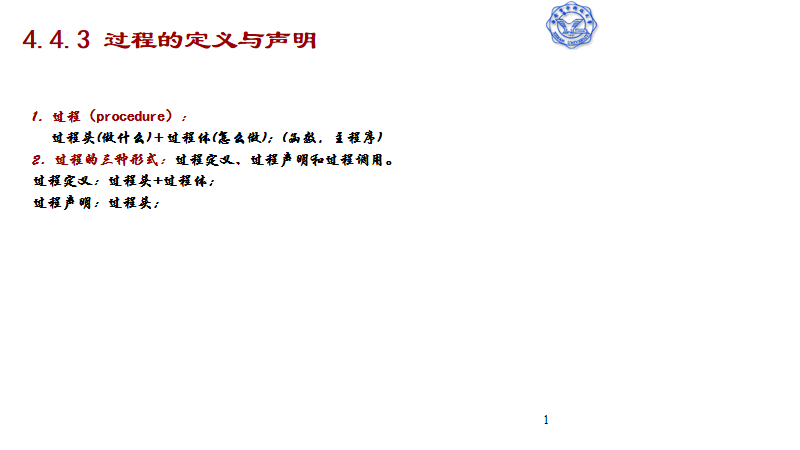左值和右值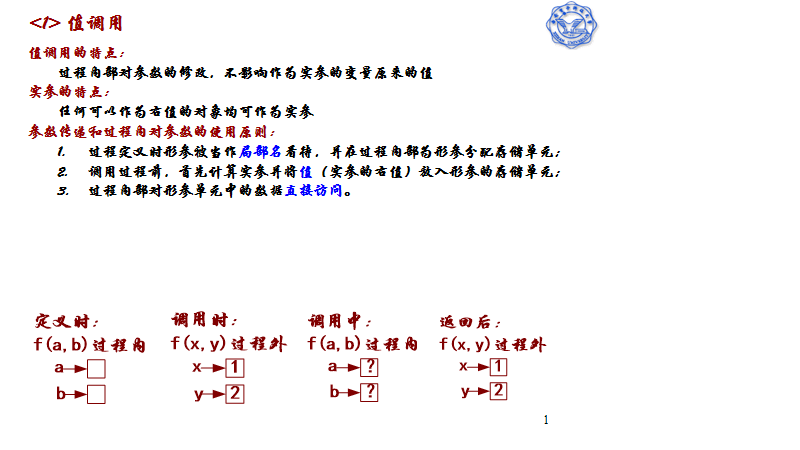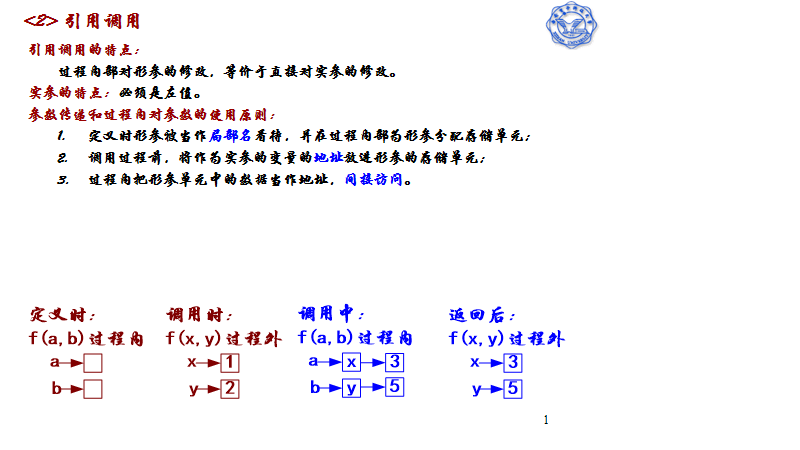左值式地址，右值是值
根据参数传递的左右值不同
调用可分为：
1.值调用    （传递右值）
2.引用调用  （传递左值）
拉链回填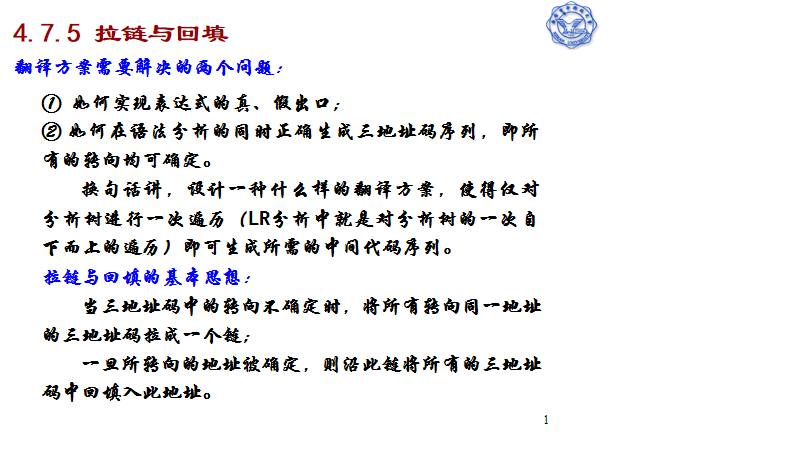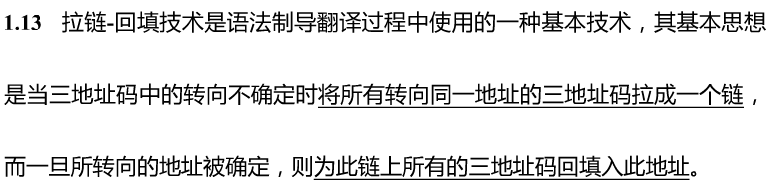展开全文• 实验报告内容要求：要给出所分析简单语言语法结构的词法说明、上下文无关文法描述，单词的种别编码方案，词法分析程序的主要算法思想，以及所采用的语法语义分析方法的算法思想的详细描述，测试结果与分析，实验总结...
• 云南大学编译技术实验报告一、实验目的 1. 进一步理解递归下降分析原理和实现方法； 2. 理解语法、语义分析程序为中心的单遍编译程序组织方法； 3. 理解语义分析的基本机制； 4. 掌握语义子程序的构造方法； 5....
云南大学编译技术实验报告

一、实验目的
1.  进一步理解递归下降分析原理和实现方法；
2.  理解语法、语义分析程序为中心的单遍编译程序组织方法；
3.  理解语义分析的基本机制；
4.  掌握语义子程序的构造方法；
5.  理解编译程序的基本逻辑过程（词法分析、语法分析、语义分析及目标代码的生成；
6.  理解编译过程中的符号表的基本管理方法；

二、实验内容
文法G[E]如下：
E→E+T | E-T |T
T→T*F | T/F | F
F→( E )
F→ id
注 id 为字母打头后面是数字或者字母的串；
在starter files基础上
1.  实现增加乘法 * 和除法 / 运算，并且，/优先级别高于+；其次，和/满足左结合规则；
2.  对包含乘除法运算的表达式生成对应的四元式；
3.  禁止同名重复声明，所以登记符号之前要检查有没有同名变量声明过；
4.  增加类型检查；

三、实验的分析与设计
1.经过修改后的翻译模式：
start->   DS.
D->B; D
D->ε
B->int L    { L.type := int }|real L    { L.type := real }
L->id   { A.Type := L.type  enter(v.entry,L.type)}  A
A-> ，idA   { A1.Type := A.type  enter(v.entry,A.type)}
A->ε
S→ V := E { gen( “:=”, E.place,0,V.place) } H
H→；S | ε
E->T    { R.i:=T.place}      R     {E.place:=R.s}
R->+T    { R1.i:= newtemp; gen( “*”, R.i, T.place , R1.i) }     R   {R.s:= R1.s; }
R-> ε    {Rs=R.i}

T→F  {p.i:=F.place}   P   {T.place:=P.s}
P->F  {p1.i:=newtemp;gen(““,P.i,T.place,P.i)}  P  {P.s:=P1.s;}
P->/F  {p1.i:=newtemp;gen(“/”,P.i,T.place,P.i)}  P  {P.s:=P1.s;}
P-> ε    {Ps=P.i}

F->( E )  { F.place := E.place}
F->id     {F.place:=position (id)}
V->id     {V.place:=position(id)}

type、i属性为继承属性，place、s属性为综合属性。

实现增加乘法 * 和除法 / 运算，并且，/优先级别高于+；其次，和/满足左结合规则；
对包含乘除法运算的表达式生成对应的四元式；
E->T    { R.i:=T.place}      R     {E.place:=R.s}
R->+T    { R1.i:= newtemp; gen( “*”, R.i, T.place , R1.i) }     R   {R.s:= R1.s; }
R-> ε    {Rs=R.i}
T→F  {p.i:=F.place}   P   {T.place:=P.s}
P->F  {p1.i:=newtemp;gen(““,P.i,T.place,P.i)}  P  {P.s:=P1.s;}
P->/F  {p1.i:=newtemp;gen(“/”,P.i,T.place,P.i)}  P  {P.s:=P1.s;}
P-> ε    {Ps=P.i}
3.  禁止同名重复声明，所以登记符号之前要检查有没有同名变量声明过；
登记符号之前要检查有没有同名变量声明过。
在enter( )这个存放声明变量的函数中，增加相应检验变量名重复的函数：

增加类型检查；
检验’:=’前后字符类型是否匹配
检验’+’前后类型是否匹配

检验’*’前后的类型是否匹配

检验’/’前后的类型是否匹配

四、实验的实现

五、运行的结果以及存在问题及其原因

#include <stdio.h>
#include<dos.h>
#include<stdlib.h>
#include<string.h>
//#include <iostream.h>
#include<ctype.h>
#include <windows.h>

#define txmax 100    /* 符号表最大容量 */
#define al 10        /* 符号的最大长度 */
#define tvmax 500    /* 最多能够申请的临时变量取值范围[100, tvmax] */
#define norw 2       /* 关键字个数 */
#define cxmax 500   /* 最多的虚拟机代码数 */

int tx;              //名字表指针, 取值范围[0, txmax-1]
int tv ;             //临时变量计数

/* 符号 */
enum symbol {
nul,         eeof,  ident,         plus,      times,    lparen,divide,
rparen,    comma,     semicolon,  becomes,  period, realsym,    intsym,
};

enum symbol sym;    /* 当前的符号 */
char ch;            /* 获取字符的缓冲区，getch 使用 */
char id[al+1];      /* 当前ident, 多出的一个字节用于存放0 */
char a[al+1];       /* 临时符号, 多出的一个字节用于存放0 */

/* 符号表结构 */
struct tablestruct
{
char name[al];      /* 名字 */
enum symbol type;   // 类型
};

struct tablestruct table[txmax]; /* 符号表 */

char word[norw][al];        /* 保留字 */
enum symbol wsym[norw];     /* 保留字对应的符号值 */
enum symbol ssym;      /* 单字符的符号值，散列表 */

int cx;             /* 四元式代码指针, 取值范围[0, cxmax-1]*/
struct instruction
{
char f[al+1]; /* 操作码 */
char l[al+1];     /* 左操作数 */
char r[al+1];     /* 右操作数*/
char t[al+1];     /* 结果 */
};
struct instruction code[cxmax]; /* 存放虚拟机代码的数组 */

FILE* fin;
FILE* fout;

int getsym();
void enter(enum symbol type);
void init();
int position(char* idt);
int gen(enum symbol op, int arg1, int arg2,int result );     //中间代码分析
void writecode(char *op, char *arg1, char *arg2,char *result );    //写缓存
void  start();
void  D();
void  B();
void L(enum symbol type);
void A(enum symbol type);
void S();
void H();
int E();
int R(int Ri);
int T();
int P(int Pi);
int F();
int V();


#include "语义分析.h"

int  main()
{
char filename;

printf("请输入分析的文件名:");
gets(filename);
if((fin=fopen(filename,"r"))==NULL)
{
printf("不能打开文件.\n");
exit(0);
}

init();                  //初始化
getsym();                //读第一个单词，将单词类别放入sym中，单词值放入id中
start();                //开始按start->DS.  分析

if (sym==eeof)
{
printf("语法正确\n\n将中间代码保存到文件请输入文件名，否则回车");
gets(filename);
if(strlen(filename)<=0) return 0;
if((fout=fopen(filename,"w"))==NULL)
{
printf("不能打开文件.\n");
exit(0);
}
for (int cx1=0; cx1<cx; cx1++)
fprintf(fout,"(%s,%s,%s,%s)\n",code[cx1].f,code[cx1].l,code[cx1].r,code[cx1].t);
return 0;
}
else
{
printf("语法错1:  . 后不能再有句子");
exit(0);
}
fclose(fin);
fclose(fout);
return 0;
}

//初始化函数
void init()
{
int i;

/* 设置单字符符号 */
for (i=0; i<=255; i++)
{
ssym[i] = nul;            //不正确的单字符符号为nul，先预置初值nul
}
ssym['+'] = plus;
ssym['*'] = times;
ssym['('] = lparen;
ssym[')'] = rparen;
ssym['.'] = period;
ssym[','] = comma;
ssym[';'] = semicolon;
ssym['/']=divide;

/* 设置保留字名字 */
strcpy(&(word), "real");
strcpy(&(word), "int");

/* 设置保留字符号 */
wsym = realsym;
wsym = intsym;

tv=100;           //临时变量指针初值，让Tx和tv的取值没有交集，区别到底是临时变变量和声明的变量
tx=0;           //表指针初值
cx=0;     //指令计数器

}

/*
* 词法分析，获取一个符号
*/
int getsym()
{
int i,k;
ch=fgetc(fin);

if (ch==EOF)
{
sym=eeof;    //文件结束
return 0;
}

while (ch==' ' || ch==10 || ch==13 || ch==9)  /* 忽略空格、换行、回车和TAB */
ch=fgetc(fin);

if (ch>='a' && ch<='z')
{
/* 名字或保留字以a..z开头 ，*/
k = 0;
do
{
if(k<al)                  /* 符号的最大长度为al ，超长就截尾处理*/
{
a[k] = ch;
k++;
}
ch=fgetc(fin);
}
while ((ch>='a' && ch<='z' )|| (ch>='0' && ch<='9'));

a[k] = 0;
strcpy(id, a);//a的地址赋予id
fseek(fin,-1,1);//文件指针偏移，向后读一个

for (i=0; i<norw; i++)      /* 搜索当前符号是否为保留字 */
if (strcmp(id,word[i]) == 0)//如果是
break;
if (i <norw)//已经经过定义，不可作为变量和过程名
{
sym = wsym[i];
}
else
{
sym = ident;          /* 搜索失败则，类型是标识符 */
}
}
else if(ch == ':') /* 检测赋值符号 */
{
ch=fgetc(fin);
if (ch == '=')
{
sym = becomes;
}
else
{
sym = nul;  /* 不能识别的符号 */
}
}
else sym = ssym[ch];     /* 当符号不满足上述条件时，全部按照单字符符号处理 */
return 0;
}

/*
* 在符号表中加入一项
*/

void enter(enum symbol type)//将声明过的变量保存在结构体table[tx]中
{
tx=tx+1;
if (tx > txmax)
{
printf(" 符号表越界 ");           /* 符号表越界 */

return;
}
for(int i=1; i<txmax; i++)
{
if(strcmp(id,table[i].name)==0)
{
printf("错误1：变量名重复定义\n");
exit(0);
}
}
strcpy(table[tx].name, id); /* 全局变量id中已存有当前名字的名字,Tx为插入当前符号之前表尾指针 */
table[tx].type = type;

}

/*
* 查找名字的位置.
* 找到则返回在名字表中的位置,否则返回0.
*
* idt:    要查找的名字
* tx:     当前名字表尾指针，全局变量
*/
/*判断是否已经存在*/
int position(char* idt)
{
int i;
strcpy(table.name, idt);
i = tx;
while (strcmp(table[i].name, idt) != 0)
{
i--;
}
return i;
}

/* 中间代码，生成四元式，目标代码*/
int gen(enum symbol op, int arg1, int arg2,int result )//通过传来的参数产生四元式
{
char temp1[al+1],temp2[al+1],temp3[al+1];
if(arg1>=100)                            //模拟申请临时变量
{
wsprintf(temp1,"T%d",arg1);//temp1是一个缓冲区，将arg1内容输出到temp1中
}
else
{
strcpy(temp1, table[arg1].name);//把table[arg1].name的内容copy到temp1中
}

if(arg2>=100)
{
wsprintf(temp2,"T%d",arg2);
}
else
{
strcpy(temp2, table[arg2].name);
}

if(result>=100)
{
wsprintf(temp3,"T%d",result);
}
else
{
strcpy(temp3,table[result].name);
}

if (op==becomes)//赋值符
{
if(table[arg1].type!=table[result].type) //检验':='前后字符类型是否匹配
{
printf("(:=,%s,%s,%s)\n",temp1,temp2,temp3);//输出四元式
writecode(":=",temp1,temp2,temp3);//写入缓存
printf("语法错：%s和%s的类型不一致\n",temp1,temp3);//':='前后字符类型不匹配，报错

}
else //:=前后字符类型匹配
{
printf("(:=,%s,%s,%s)\n",temp1,temp2,temp3);
writecode(":=",temp1,temp2,temp3);//写入缓存

}
}

else if (op==plus)                          //+运算
{

if(table[arg1].type==table[arg2].type)//检验'+'前后类型是否匹配
{
table[result].type=table[arg1].type;
writecode("+",temp1,temp2,temp3);
printf("(+,%s,%s,%s)\n",temp1,temp2,temp3);
}

else
{
printf("(+,%s,%s,%s)\n",temp1,temp2,temp3);
writecode("+",temp1,temp2,temp3);
printf("语法错：%s和%s的类型不一致\n",temp1,temp2);
}
}

/*乘法*/
else if(op==times)
{
if(table[arg1].type==table[arg2].type)//检验'*'前后的类型是否匹配
{
table[result].type=table[arg1].type;
printf("(*,%s,%s,%s)\n",temp1,temp2,temp3);
writecode("*",temp1,temp2,temp3);
}
else
{
printf("(*,%s,%s,%s)\n",temp1,temp2,temp3);
writecode("*",temp1,temp2,temp3);
printf("语法错：%s和%s的类型不一致\n",temp1,temp2);

}

}

/*除法*/
else if(op==divide)
{
if(table[arg1].type==table[arg2].type)//检验'/'前后的类型是否匹配
{
table[result].type=table[arg1].type;
printf("(/,%s,%s,%s)\n",temp1,temp2,temp3);
writecode("/",temp1,temp2,temp3);
}
else
{
printf("(/,%s,%s,%s)\n",temp1,temp2,temp3);
writecode("/",temp1,temp2,temp3);
printf("语法错：%s和%s的类型不一致\n",temp1,temp2);

}
}
return 0;
}

//处理代码段，打印
void writecode(char op[al+1], char arg1[al+1], char arg2[al+1],char result[al+1] )
{
if (cx >= cxmax)
{
printf("Program too long"); /* 程序过长 */
return ;
}
strcpy(code[cx].f, op);
strcpy(code[cx].l,arg1);
strcpy(code[cx].r,arg2);
strcpy(code[cx].t,result);
cx++;
return ;
}

/*分析产生式     X->DS.   */
void  start()
{
if (sym==intsym||sym==realsym)//如果变量是int或者real型
{
D();
S();
if (sym==period)
{
getsym();//调用词法分析
return;
}
else
{
printf("语法错2： 缺少程序结束.");
exit(0);
}
}
else
{
printf("语法错3: 程序只能用int,和real开始，而且区分大小写");
exit(0);
}
}

/*递归下降分析
D->  B; D
D->ε

*/
void  D()
{
if (sym==intsym ||sym==realsym)
{
B();
if (ch=';')
{
getsym();
D();
}
else
{
printf("语法错4");
exit(0);
}
}
else if(sym==ident)  return;
else
{
printf("语法错5");
exit(0);
}
}

/*
B->   int L    { L.type := int }|real L    { L.type := real }
*/
void  B()
{
if (sym==intsym )//继承L的类型
{
getsym();
L(intsym);
}
else if (sym==realsym)
{
getsym();
L(realsym);
}
else
{
printf("语法错6：变量不是real或int型\n");
exit(0);
}
}

/*
L->   id   { A.Type := L.type  enter(v.entry,L.type)}   A       V.entry通过全局变量tx隐性传递
有参，有几个继承属性就有几个参数
*/
void L(enum symbol type)//L的id赋予A
{
int Vplace;
if (sym==ident)
{
Vplace=position(id);
if(Vplace>0)
{
printf("语法错7：变量重复声明\n");
exit(0);
}
enter(type);
getsym();
A(type);
}
else
{
printf("语法错8：缺少变量");
exit(0);
}
}

/*
A-> ，id  A   { A1.Type := A.type  enter(v.entry,A.type)}
A->ε
*/

void A(enum symbol type)
{
int Vplace;
if (sym==comma)          //当前单词为，
{
getsym();
if (sym==ident)
{
Vplace=position(id);
if(Vplace>0)
{
printf("语法错7：变量重复声明\n");
exit(0);
}
enter(type);
getsym();
A(type);
}
else
{
printf("语法错9");
exit(0);
}
}
else if (sym== semicolon)   return ;//当前单词为；即A的follow集元素相当于进行A->ε
else
{
printf("语法错10");
exit(0);
}
}

/*
S→ V := E { gen( ":=", E.place,0,V.place) } H
*/
void S()
{
int vplace,Eplace;
if (sym==ident)
{
vplace=V();
//getsym();
if (sym==becomes)     //当前单词为:=
{
getsym();
Eplace=E();
gen(becomes,Eplace,-1,vplace);
H();
}
else
{
printf("语法错11");
exit(0);
}
}
else
{
printf("语法错12");
exit(0);
}
}

/*
H→；S | ε
*/
void H()//执行下一条运算
{
if (sym==semicolon)          //当前单词为indent类型
{
getsym();
S();
}
else if (sym==period)   return ;
else
{
printf("语法错13");
exit(0);
}
}
/*
E->T      { R.i:=T.place}      R     {E.place:=R.s}
综合属性，有返回值
*/

int E()//分析赋值号后面的运算
{
int ri,tplace,Rs;
if (sym==ident || sym== lparen)//当前为标识符或左括号
{
tplace=T();
ri=tplace;
Rs=R(ri);
}
else
{
printf("语法错14");
exit(0);
}
return Rs;
}

/*
R->+T     { R1.i:= newtemp; gen( "*", R.i, T.place , R1.i) }     R   {R.s:= R1.s; }
R-> ε    {R.s=R.i}
R既有参数又有返回值
*/

int R(int Ri)//检验+运算
{
int Rs,tplace;
if (sym==plus)
{
getsym();
tplace=T();
tv=tv+1;            //生成临时变量
gen(plus,Ri,tplace,tv);
Rs=R(tv);
}
else if (sym== semicolon || sym==rparen|| sym==period)
{
Rs=Ri;
}
else
{
printf("语法错15");
exit(0);
}
return Rs;
}

/*实现乘除的左结合及优先级
F->( E )  { F.place := E.place}
F->id     {F.place:=position (id)}
*/
int F()//实现*、/运算的优先级高于+运算
{
int Fplace;
if(sym==ident)
{
Fplace=position(id);//获取当前位置
if(Fplace==0)
{
printf("变量没有声明\n");
exit(0);
}
getsym();
}
else if(sym==lparen)
{
getsym();
Fplace=E();
if(sym==rparen)getsym();
else
{
printf("语法错16，缺)");
exit(0);
}
}

else
{
printf("语法错17,，缺(");
exit(0);
}
return Fplace;
}

/*
P->*F  {p1.i:=newtemp;gen("*",P.i,T.place,P.i)}  P  {P.s:=P1.s;}
P->/F  {p1.i:=newtemp;gen("/",P.i,T.place,P.i)}  P  {P.s:=P1.s;}
P-> ε    {Ps=P.i}
*/
int P(int Pi)//检验*、/运算
{
int ps,Fplace;
if(sym==times)
{
getsym();
Fplace=F();
tv=tv+1;//生成临时变量
gen(times,Pi,Fplace,tv);
ps=P(tv);
}

else if(sym==divide)
{
getsym();
Fplace=F();
tv=tv+1;
gen(divide,Pi,Fplace,tv);
ps=P(tv);
}

else if(sym==plus||sym==semicolon||sym==rparen||sym==period)
//求出P的follow集，进行语法检查，如判断左括号是否匹配
{
ps=Pi;
}

else
{
printf("语法错18：缺少变量或(");
exit(0);
}

return ps;
}
/*
将T的位置指向新增加乘除
T→F  {p.i:=F.place}   P   {T.place:=P.s}
*/
int T()
{
int Pi,Fplace,Ps;
if(sym==ident||sym==lparen)
{
Fplace=F();
Pi=Fplace;
Ps=P(Pi);
}

else
{
printf("语法错19");
exit(0);
}
return Ps;
}

/*

V->id     {V.place:=position(id)}
*/
int V()
{
int Vplace;
if (sym==ident)
{
Vplace=position (id);
if (Vplace==0)
{
printf("变量没有声明");
exit(0);
}
getsym();
}
else
{
printf("语法错20");
exit(0);
}
return Vplace;
}

展开全文pl0编译器
• 语义分析： （见语法制导翻译篇） 语义是指源程序及其组成部分所表述的含义 和语法不同，语法是关于程序及其组成部分的构成规则的描述，是上下文无关的；而语义是关于语法结构的含义及其使用规则的描述，是上下文...
语义分析：
（见语法制导翻译篇）

语义是指源程序及其组成部分所表述的含义
和语法不同，语法是关于程序及其组成部分的构成规则的描述，是上下文无关的；而语义是关于语法结构的含义及其使用规则的描述，是上下文有关的。
语法上正确，其语义不一定正确。语义分析与中间代码生成器基于语义规则，对语法树进行语义分析（变量是否定义，类型是否正确）和中间代码生成（三元式、四元式等）。 主要功能包括建立符号表，进行静态语义检查，发现语义错误
编译器分析的语义都是静态语义，静态语义是指在编译器间可以确定的语义，与之对应的动态语义只能在运行期间才能被确定。
静态语义分析通常包括声明、类型匹配、类型转换等。经过语义分析之后，在语法分析生成的语法树的基础上进一步对表达式做一些标识。如：有些某些类型需要做隐式转化，语义分析器会在之前的语法树中插入相应的转换节点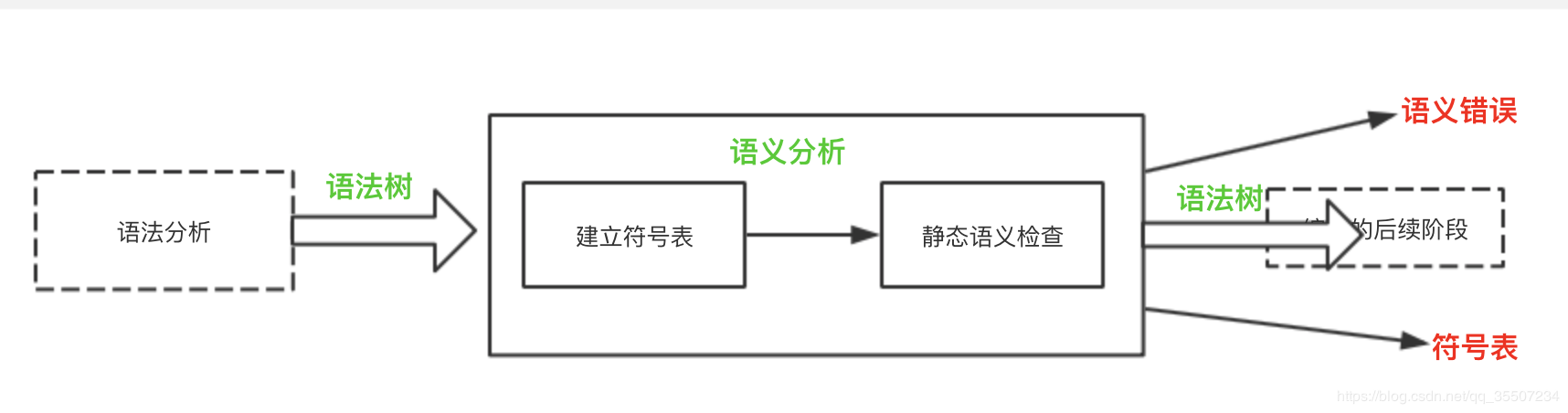2.语义描述
程序的语义，函数的语义，各种名字的声明和使用的语义， 各种语句的语义，表达式的语义
3.符号表
在编译程序工作的过程中，需要不断收集、记录和使用源程序中的各种名字及其属性等相关信息，以便检查语义是否正确，并辅助翻译为正确的代码。一般是建立表格的方式记录信息。
功能1）收集符号信息  2）进行语义的合法性检查
符号表应该包含常量表、变量表、函数表等等。
常见的语义错误主要有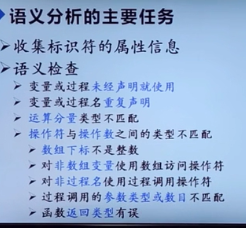展开全文• 编译原理实验报告：语义分析及中间代码生成1. 实验题目：语义分析及中间代码生成实验目的实验内容实验要求输入输出2. 设计思想3.算法流程4. 源程序5. 调试数据 1. 实验题目：语义分析及中间代码生成 实验目的 通过...
编译原理实验：语义分析及中间代码生成1. 实验题目：语义分析及中间代码生成实验目的实验内容实验要求输入输出2. 设计思想3.算法流程4. 源程序5. 调试数据
1. 实验题目：语义分析及中间代码生成
实验目的

通过上机实习，加深对语法制导翻译原理的理解，掌握将语法分析所识别的语法范畴变换为某种中间代码的语义翻译方法。
掌握目前普遍采用的语义分析方法──语法制导翻译技术。
给出PL/0文法规范，要求在语法分析程序中添加语义处理，对于语法正确的表达式，输出其中间代码；对于语法正确的算术表达式，输出其计算值。

实验内容
已给PL/0语言文法，在实验二或实验三的表达式语法分析程序里，添加语义处理部分，输出表达式的中间代码，用四元式序列表示。
实验要求

语义分析对象重点考虑经过语法分析后已是正确的语法范畴，本实验重点是语义子程序。
在实验二或实验三“语法分析器”的里面添加PL/0语言“表达式”部分的语义处理，输出表达式的中间代码，计算表达式的语义值。
中间代码用四元式序列表示。

输入输出

PL/0算术表达式的语义计算：
输入：
PL/0算术表达式，例如: 2 + 3 * 5作为输入。
输出：
17
PL/0表达式的中间代码表示
输入：
PL/0表达式，例如: a *（b +c）
输出：
( + b  c  t1 )
( * a  t1  t2 )

2. 设计思想
本次实验我采用的递归下降分析器的设计。
递归下降分析法的原理是利用函数之间的递归调用来模拟语法树自上而下的构建过程。从根节点出发，自顶向下为输入串中寻找一个最左匹配序列，建立一棵语法树。在不含左递归和每个非终结符的所有候选终结首字符集都两两不相交条件下，我们就可能构造出一个不带回溯的自顶向下的分析程序，这个分析程序是由一组递归过程（或函数）组成的，每个过程（或函数）对应文法的而一个非终结符。
语法：
<表达式> -> [+|-]<项>{<加法运算符> <项>}
<项> -><因子>{<乘法运算符> <因子>}
<因子> -> <标识符>|<无符号整数>|(<表达式>)
<加法运算符> -> +|-
<乘法运算符> -> *|/
计算FIRST集：
FIRST(<表达式>)={ +， -， (， <标识符>， <无符号整数> }
FIRST(<因子>)={ <标识符>， <无符号整数>， ( }
FIRST(<项>)={ <标识符>， <无符号整数>， ( }
FIRST(<加法运算符>)={ +， - }
FIRST(<乘法运算符>)={ *， / }
计算FOLLOW集：
FOLLOW(<表达式>)={ ) }
FOLLOW (<加法运算符>)={ <标识符>， <无符号整数>， ( }
FOLLOW (<乘法运算符>)={ <标识符>， <无符号整数>， ( }
可以改成如下拓广文法：
<表达式>-><项> | <表达式>+<项> | <表达式>-<项>
<项>-><因子> | <项>*<因子> | <项>/<因子>
<因子>->(<表达式>) | <标识符> | <无符号整数>
将语法消除左递归可得(用E代表<表达式>，T代表<项>，F代表<因子>)
E->TE’
E’->ɛ|+TE’|-TE’
T->FT’
T’->ɛ|*FT’|/FT’
F->ident|(E)|number
属性文法：是在上下文无关文法的基础上为每个文法符号（终结符或非终结符）配备若干个相关的“值”（称为属性）。
属性:代表与文法符号相关的信息，和变量一样，可以进行计算和传递。
综合属性
用于“自下而上”传递信息
在语法树中，一个结点的综合属性的值，由其子结点的属性值确定
S—属性文法：仅仅使用综合属性的属性文法
语义规则： 属性计算的过程即是语义处理的过程
对于文法的每一个产生式配备一组属性的计算规则，则称为语义规则。
(1)终结符只有综合属性，它由词法分析器提供
(2)非终结符既可以有综合属性也可以有继承属性，文法开始符号的所有继承属性作为属性计算前的初始值。
(3) 产生式右边符号的继承属性和产生式左边符号的综合属性都必须提供一个计算规则
(4) 产生式左边符号的继承属性和产生式右边符号的综合属性不由所给的产生式的属性计算规则进行计算，它们由其它产生式的属性规则计算
一遍扫描的处理方法： 与树遍历的属性计算方法不同，一遍扫描的处理方法是在语法分析的同时计算属性值，而不是语法分析构造语法树之后进行属性的计算，而且无需构造实际的语法树。
因为一遍扫描的处理方法与语法分析器的相互作用，它与下面两个因素密切相关：
1.所采用的语法分析方法
2.属性的计算次序
如下为递归下降分析器的设计实现思想

对每个非终结符A构造一个函数过程，对A的每个继承属性设置一个形式参数，函数的返回值为A的综合属性（作为记录，或指向记录的一个指针，记录中有若干域，每个属性对应一个域）。
非终结符A	对应的函数过程中，根据当前的输入符号决定哪个产生式候选。
每个产生式对应的代码中，按照从左到右的次序，对于单词符号（终结符），非终结符和语义分析分别做以下的工作。
（1）对于带有综合属性x的终结符X，把x的值存入为X.x设置的变量中。然后产生一个匹配X的调用，并继续读入一个输入符号。
（2）对于每个非终结符号B，产生一个右边带有函数调用的赋值语句c=B(b1,b2,…，bk)
（3）对于语义动作，把动作的代码抄进分析器中，用代表属性的变量来代替对应属性的每一次引用。

3.算法流程

每一个非终结符对应于一个函数（子过程）；
非终结符所对应的右侧产生式为函数体；
每遇到一个终结符，则需要判断所输入字符是否与之匹配，若匹配则读取下一个，若不匹配，则进行出错处理。
算法过程：

PROCEDURE <表达式>:
BEGIN
IF SYM=’+’ OR SYM=’-’ THEN
BEGIN
WHILE SYM=’+’ OR SYM=’-’ DO
BEGIN
END
END
ELSE IF SYM=FIRST(<项>) THEN
BEGIN
<项>;
WHILE SYM=’+’ OR SYM=’-’ DO
BEGIN
END
END
ELSE ERROR
END

PROCEDURE <项>:
BEGIN
IF SYM=’*’ OR SYM=’/’ THEN
BEGIN
WHILE SYM=’*’ OR SYM=’/’ DO
BEGIN
END
END
ELSE IF SYM=FIRST(<因子>) THEN
BEGIN
<因子>;
WHILE SYM=’*’ OR SYM=’/’ DO
BEGIN
END
END
ELSE ERROR
END

PROCEDURE <因子>:
BEGIN
IF SYM=’标识符’ OR SYM=<无符号整数> THEN
BEGIN
END
ELSE IF SYM=’(’ THEN
BEGIN
<表达式>
IF SYM=’)’ THEN
BEGIN
END
ELSE ERROR
END
ELSE ERROR
END

PROGRAM PAESER
BEGIN
<表达式>；
IF SYM<>’#’ THEN ERROR
END

因此要在如上的基础上构造函数过程，函数中会包含计算属性。
4. 源程序
#include<bits/stdc++.h>
using namespace std;
ifstream infile("F:\\编译原理\\第四次实验\\result.txt");//文件流
ifstream infile2("F:\\编译原理\\第四次实验\\analysis.txt");//文件流
ofstream outfile("F:\\编译原理\\第四次实验\\result.txt");//文件输出流
map<string,string> word;//应用map数据结构形成一个string->string的对应
std::map<string,string>::iterator it;//用来遍历整个对应关系的迭代器
string str;//读入的字符串
string sym;//用来指示读入的符号
string sym2;//用来指示读入的符号
int count1=0,k=0,flag=0,conterr=0,lpnum=0;
string expressionAnalysis();//表达式分析，表达式的中间代码表示
string termAnalysis();//项分析，表达式的中间代码表示
string factorAnalysis();//因子分析，表达式的中间代码表示
int expressionAnalysis2();//表达式分析，算数表达式的语义计算
int termAnalysis2();//项分析，算数表达式的语义计算
int factorAnalysis2();//因子分析，算数表达式的语义计算
string result;
string arg1;
string arg2;
string op;
};
void map_init(){//对应关系进行初始化，如下只包括了表达式的相关符号
word["+"]="plus";
word["-"]="minus";
word["*"]="times";
word["/"]="slash";
word["="]="eql";
word["("]="lparen";
word[")"]="rparen";
}
void lexanalysis(){//词法分析
char ch;
char a;
string word1;//string变量识别单词
string str;//string变量进行字符识别
ostringstream buf;
while(buf&&infile2.get(ch)) buf.put(ch);//将文件中的字符读出来
str= buf.str();//将得到的字符储存到string类型变量中
int csize=str.length();
for(int i=0;i<csize;i++){//对整个字符串进行遍历
while(str[i]==' '||str[i]=='\n') i++;//若最开始为空格或换行符，则将指针的位置往后移
if(isalpha(str[i])){//对标识符和基本字进行识别,调用库函数isalpha()
word1=str[i++];
while(isalpha(str[i])||isdigit(str[i])){
word1+=str[i++];
}
it=word.find(word1);
if(it!=word.end()){//判断是不是基本字，若为基本字则进行输出
outfile<<"("<<word[word1]<<","<<word1<<")"<<endl;
}
else{//否则直接输出
outfile<<"(ident"<<","<<word1<<")"<<endl;
}
i--;
}
else if(isdigit(str[i])){//判断是不是常数，调用库函数isdigit()
word1=str[i++];
while(isdigit(str[i])){
word1+=str[i++];
}
if(isalpha(str[i])){
outfile<<"error"<<endl;
break;
}
else{
outfile<<"(number"<<","<<word1<<")"<<endl;
}
i--;
}else{//对其他的基本字依次进行判断
word1=str[i];
it=word.find(word1);
if(it!=word.end()){
outfile<<"("<<word[word1]<<","<<word1<<")"<<endl;
}else{
outfile<<"error"<<endl;
break;
}
}
}
infile2.close();
}
int found1,found2;
if(!getline(infile,str)){
return 0;
}
found1=str.find(',',0);
if(found1==-1){
conterr++;
cout<<"语法错误 识别字符错误"<<endl;
return -1;
}
found2=str.length();
sym=str.substr(1,found1-1);
sym2=str.substr(found1+1,found2-found1-2);
return 1;
}
string newtemp(){//产生新变量名t1,t2等
char *p;
char m;
p=(char*)malloc(12);
k++;
itoa(k,m,10);
strcpy(p+1,m);
p='t';
string s;
s=p;
return s;
}
void emit(string op,string arg1,string arg2,string result){//产生四元式用于显示
count1++;
return;
}
string expressionAnalysis(){//表达式的递归下降分析程序
string op,arg1,arg2,result;
if(conterr){
return NULL;
}
arg1=termAnalysis();//通过项分析得到第一个参数的值
if(conterr){
return NULL;
}
while((sym=="plus")||(sym=="minus")){
op=sym2;
if(conterr){
return NULL;
}
if(flag==0){
cout<<"语法错误 <加法运算符>后缺项"<<endl;
conterr++;
return NULL;
}
arg2=termAnalysis();//通过项分析得到第二个参数的值
if(conterr){
return NULL;
}
result=newtemp();//产生中间变量名，相当于对结果进行存储
emit(op,arg1,arg2,result);//产生四元式，相当于进行加法或减法运算，得出结果
arg1=result;//保存得到的结果
}
return arg1;//返回表达式最终得到的值
}
string termAnalysis(){//项的递归下降分析程序
string op,arg1,arg2,result;
arg1=factorAnalysis();//通过因子分析得到第一个参数的值
if(conterr){
return NULL;
}
while((sym=="times")||(sym=="slash")){
op=sym2;
if(conterr){
return NULL;
}
if(flag==0){
conterr++;
cout<<"语法错误 <乘法运算符>后缺因子"<<endl;
return NULL;
}
if(conterr){
return NULL;
}
arg2=factorAnalysis();//通过因子分析得到第二个参数的值
if(conterr){
return NULL;
}
result=newtemp();//产生中间变量名，相当于对结果进行存储
emit(op,arg1,arg2,result);//产生四元式，相当于进行乘法或除法运算，得出结果
arg1=result;//保存得到的结果
}
return arg1;//返回项最终得到的值
}
string factorAnalysis(){
string arg;
if(sym=="ident"){//如果是标识符，最终返回标识符的符号
arg=sym2;
if(conterr){
return NULL;
}
if(lpnum==0&&sym=="rparen"){
conterr++;
cout<<"语法错误 ')'不匹配"<<endl;
return NULL;
}
}
else if(sym=="number"){//如果是无符号整数，最终返回相应的整数
arg=sym2;
if(conterr){
return NULL;
}
if(lpnum==0&&sym=="rparen"){
conterr++;
cout<<"语法错误 ')'不匹配"<<endl;
return NULL;
}
}
else if(sym=="lparen"){//如果是左括号，则要进行右括号匹配，并判断中间是不是表达式，并得出表达式的值进行返回
lpnum++;
if(conterr){
return NULL;
}
if(flag==0){
conterr++;
cout<<"语法错误 '('后缺少表达式"<<endl;
return NULL;
}
arg=expressionAnalysis();
if(conterr){
return NULL;
}
if(flag==0||sym!="rparen"){
conterr++;
cout<<"语法错误 表达式后面缺少')'"<<endl;
return " ";
}else{
lpnum--;
if(conterr){
return NULL;
}
if(flag==0){
return arg;
}
}
}else{
cout<<"语法错误 因子首部不为<标识符>|<无符号整数>|'('"<<endl;
conterr++;
return " ";
}
return arg;
}
int expressionAnalysis2(){//表达式的递归下降分析程序
string op;
int arg1,arg2,result;
if(conterr){
return 0;
}
arg1=termAnalysis2();//通过项分析得到第一个参数的值
if(conterr){
return 0;
}
while((sym=="plus")||(sym=="minus")){
op=sym2;
if(conterr){
return 0;
}
if(flag==0){
cout<<"语法错误 <加法运算符>后缺项"<<endl;
conterr++;
return 0;
}
arg2=termAnalysis2();//通过项分析得到第二个参数的值
if(conterr){
return 0;
}
if(op=="+"){//若是加法符号则进行加法运算，并对得到的结果进行保存
result=arg1+arg2;
arg1=result;
}
else{//若是减法符号则进行加法运算，并对得到的结果进行保存
result=arg1-arg2;
arg1=result;
}
}
return arg1;//返回该表达式所代表的值
}
int termAnalysis2(){//项的递归下降分析程序
string op;
int arg1,arg2,result;
arg1=factorAnalysis2();//通过因子分析得到第一个参数的值
if(conterr){
return 0;
}
while((sym=="times")||(sym=="slash")){
op=sym2;
if(conterr){
return 0;
}
if(flag==0){
conterr++;
cout<<"语法错误 <乘法运算符>后缺因子"<<endl;
return 0;
}
if(conterr){
return 0;
}
arg2=factorAnalysis2();//通过因子分析得到第二个参数的值
if(conterr){
return 0;
}
if(op=="*"){//若是乘法符号则进行加法运算，并对得到的结果进行保存
result=arg1*arg2;
arg1=result;
}
else{//若是除法符号则进行加法运算，并对得到的结果进行保存
if(arg2==0){
conterr++;
cout<<"除数不能为0"<<endl;
return 0;
}
result=arg1/arg2;
arg1=result;
}
}
return arg1;//返回该项所代表的值
}
int factorAnalysis2(){
int arg;
if(sym=="ident"){//算数表达式中不含有字母，否则无法进行运算
cout<<"算术表达式中含有字母"<<endl;
conterr++;
return 0;
}else if(sym=="number"){//若果是数字，则返回相应的值
arg=atoi(sym2.c_str());
if(conterr){
return 0;
}
if(lpnum==0&&sym=="rparen"){
conterr++;
cout<<"语法错误 ')'不匹配"<<endl;
return 0;
}
}
else if(sym=="lparen"){//如果是左括号，则要进行右括号匹配，并判断中间是不是表达式，并得出表达式的值进行返回
lpnum++;
if(conterr){
return 0;
}
if(flag==0){
conterr++;
cout<<"语法错误 '('后缺少表达式"<<endl;
return 0;
}
arg=expressionAnalysis2();//返回表达式的值
if(conterr){
return 0;
}
if(flag==0||sym!="rparen"){
conterr++;
cout<<"语法错误 表达式后面缺少')'"<<endl;
return 0;
}else{
lpnum--;
if(conterr){
return 0;
}
if(flag==0){
return arg;
}
}
}else{
cout<<"语法错误 因子首部不为<标识符>|<无符号整数>|'('"<<endl;
conterr++;
return 0;
}
return arg;//返回该因子所代表的值
}
int main(){
int i=0,num,result;
//开始词法分析
map_init();
lexanalysis();
//开始语法和语义分析
cout<<"1.PL/0表达式的中间代码表示"<<endl;
cout<<"2.PL/0算术表达式的语义计算"<<endl;
cout<<"请输入类别号码:";
cin>>num;
if(num==1){//PL/0表达式的中间代码表示
if(flag){
expressionAnalysis();//开始进行表达式分析
}
if(flag!=-1&&!conterr){//若表达式正确则输出表达式的中间代码表示
for(int i=0;i<count1;i++){
}
}
}else if(num==2){//PL/0算术表达式的语义计算
if(flag){
result=expressionAnalysis2();//开始进行表达式分析
}
if(flag!=-1&&!conterr){//若表达式正确则输出表达式的值
cout<<result<<endl;
}
}else{
cout<<"error!"<<endl;
return 0;
}
infile.close();
return 0;
}

5. 调试数据
待输入的文件流：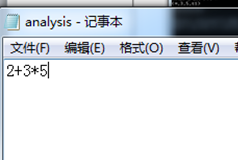词法分析结果：最终得到的结果：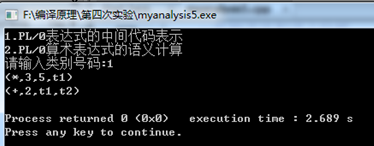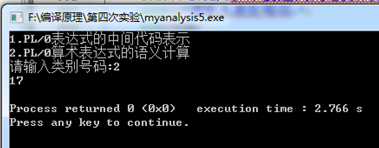待输入的文件流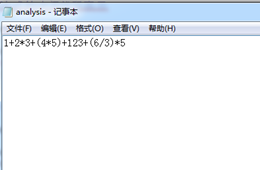词法分析结果：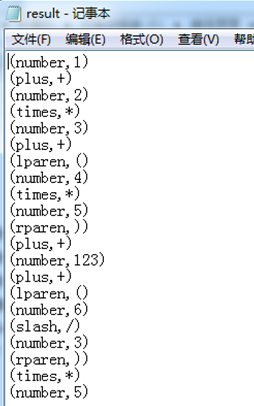最终得到的结果：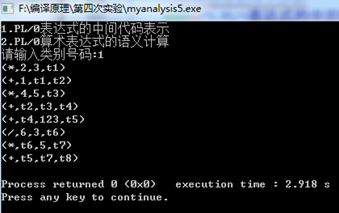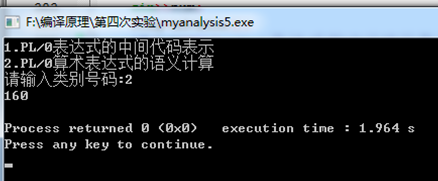展开全文• 语义分析的必要性 语法和语义的区别 语法关于什么样的字符串才是该语言 在组成结构上合法的程序的法则 语义关于结构上合法的程序的意义的 法则 语义分析的分类 语义种类 静态语义在编译阶段(从程序文本上)可 以检查...
• 实验目的：用c语言对一个简单语言的子集编制一个一遍扫描的编译程序，以加深对编译原理的理解,掌握编译程序的实现方法和技术。 语法分析 C2.1 实验目的 编制一个递归下降分析程序,实现对词法分析程序所提供的单词...
• 实验五 语义分析器 代码已开源：https://github.com/LinXiaoDe/Quary/tree/master/lab5 一. 学习经典的语义分析器（2小时） 一、实验目的 学习已有编译器的经典语义分析源程序。 二、实验任务 阅读已有编译器的...
• 语义分析的作用 检查结构正确的句子表达的意思是否正确 执行规定的语义动作 中间代码简介 后缀式 逆波兰式，a+bc的后缀式表示为abc+操作符紧跟在操作数的后面 三地址码 三地址码式不超过三个地址...
•  语义分析编译的第三步，它的主要任务是：1.收集标识符的属性信息信息，如收集种属信息（简单变量、复合变量（数组等）、过程），类型信息，存储位置，长度，值，作用域，参数，返回值等。在语义分析阶段收集的...
• 下列哪项工作属于语义分析的范畴? A、 单词拼写错误检查 B、 括号不匹配 C、 加法运算两个操作数的类型不兼容 D、 寄存器分配 正确答案： C 我的答案：C 得分： 3.5分 2 程序设计语言的语义分为静态语义和动态语义，...编译器
• 文章目录第一节 语义分析概述1.1 语义分析的任务1.2 语义分析和语法分析1.3 语义值1.4 语义动作和语义子程序1.5 翻译1.6 三地址码第二节 说明语句的翻译第三节 赋值语句的翻译第四节 goto语句的翻译第五节 if语句的...编程语言
• 编译原理实践：C++实现语义分析器（学习笔记）实践说明输入举例（input.txt）输出举例（output.txt）编程平台代码实现基本思路语义分析部分预定义主函数定义语义分析部分函数语法分析主函数语句串分析函数语句分析...c++ 编译器
• 　这次实验的实验目的其实很明确——对源码进行语义分析，输出语义分析结果，并要求有适当的错误处理机制。可是指导书上实验目的要求自己分析，我的分析结果：本次实验要求自己定义上次实验的语法分析的文法的SDD，...java Java JAVA
• 第七章 语义分析和中间代码的产生一·内容小结 语义分析和中间代码的产生式编译程序的第三个步骤，它将对语法分析所识别的各类语法范畴，分析其含义，并进行初步翻译，产生中间代码。这一章主要有以下内容：中间...
• 根据对属性文法及语义分析、中间代码生成的学习，可以将实验二、三的两种语法分析器进行一定的改造，以达到进行语法分析的同时进行语义分析并生成中间代码。根据PL0文法的特点以及尝试进行一次语法分析完成语义分析...编译器 c++ 算法
• 若仅有一个孩子，则nptr2为空 函数mkleaf(node)： 生成一个叶子节点 产生式： 语义规则： (1) A → id := E {A.nptr:= mknode(:=,mkleaf(entry(id.name)),E.nptr)} (2) E → E1 + E2 {E.nptr:=mknode(+,E1....
• 2017计科01-08编译原理练习题一语义分析&中间代码生成 单选题 2-1中间代码生成所依据的是（ C ）。 A.语法规则 B.词法规则 C.语义规则 D.等价变换规则 2-2四元式之间的联系是通过（ B ）实现的。 A.指示器 B....中间代码
• 这是语义分析的说明统计，可以看一下啊sfawefasdfsdf
• 语义分析 编译原理 北邮 大三 实验要求：编写语义分析程序，实现对算术表达式的类型检查和求值。要求所分析算术表达式由如下的文法产生。 实验要求：用自底向上的语法制导翻译技术实现对表达式的分析和翻译。 (1) ...
• 第7章 语义分析与中间代码生成 重点：三地址码，各种语句的目标代码结构、 语法制导定义与翻译模式。 难点：布尔表达式的翻译，对各种语句的目标  代码结构、语法制导定义与翻译模式的  理解。 7.1 中间...
• (2) 掌握目前普遍采用的语义分析方法──语法制导翻译技术。 (3) 给出 PL/0 文法规范，要求在语法分析程序中添加语义处理，对于语 法正确的表达式，输出其中间代码；对于语法正确的算术表达式， 输出其计算值。 2. ...c++
• 注：本文记录　WHUT-计算机学院-编译原理　课程　课内实践 ... 纸上得来终觉浅，觉知此事需躬行！ 0、笔者： 编译原理课程一共有两次实验和一次课内... 赋值语句的语义分析程序设计与实现 而课内实践的内容是：WHI...赋值语句
• 第1章 绪论 第2章 指令系统 第3章 可执行文件 第4章 反汇编技术 第5章 指令的语义抽象 第6章 基本数据类型分析 第7章 高级控制流恢复 第8章 过程恢复技术 第9章 部分编译优化效果的消除 第10章 程序的调试与测试
• 概述 读一段程序和读一篇文章的处理是有相似之处的 首先需要能够认出一个个字符（字，单词或者标点） ...**但是编译的目的是让计算机或者运行环境（而不是人脑）理解程序的含义，所以语义分析的阶段就特别困难，因为...
• 语义分析做了哪几件事？这几件事分别通过什么方法完成的？最后的结果是什么？ 1. java类中的符号输入到符号表；（添加默认的构造器） 过程：即把抽象语法树中符号输入到符号表中，这里的符号包括import节点上的符号...javac
• 摘要：编译原理简介，反编译原理简介，反编译的主要步骤，反编译的意义。...欲理解反编译技术的原理所在，我们需要先系统的了解一下编译技术编译技术就是把高级语言变成可执行文件的过程。它的主要过程...